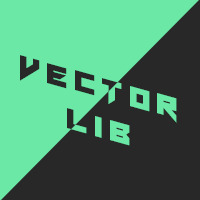# VectorLib

#### Gizmo199

You must be logged in to obtain assets

### Description

Vector-library A Vector2 and Vector3 library for Game Maker Studio 2

This is a full vector library for Game Maker Studio 2! This is an ongoing and growing project so keep up-to-date on new updates!

Declaration Using them is super easy! just use the 'new' command and choose either a vec2 or vec3! Example:

``````my_vec = new vec2(0, 0); // This sets a new vector2 with the values x=0, y=0
// OR
my_vec = new vec2(0);    // This also sets a new vector2 with the values x=0, y=0
``````

You can use the same method with vec3's but also use vec2's in them!

``````my_vec2 = new vec2(1);          // This creates a vector 2 with values x=1, y=1
my_vec3 = new vec3(my_vec2, 0); // This creates a vector 3 with values x=1, y=1, z=0
``````

Variables Both vectors contains an x and y value. Vec3's also contain a z, xy, and yx value! xy will return a vector 2 of x and y ( eg. vec2(x,y) ) yx will return a vector 2 of y and x ( eg. vec2(y,x) )

Functions Both vectors contain:

• subtract
• multiply
• divide
• modulo
• length_squared
• length
• normalize
• is_normalized
• inverse
• dot
• dot_normalized
• distance_to
• angle_to
• lerp_to
• clamp_to
• reflect
• vec_abs ( works like abs() )
• vec_ceil ( works like ceil() )
• vec_sign ( works like sign() )
• vec_round ( works like round() )
• vec_floor ( works like floor() ) Vec3's have an added function called 'Cross()' which returns the cross product of 2 vector 3s

Chained Functions Functions can be chained together like so:

``````my_vec = new vec3(1);
``````

This will make the vector my_vec equal x=4, y=4, z=4 because:

``````.add(1) = 1+1, 1+1, 1+1 = ( x=2, y=2, z=2 )
.multiply(2) = 2*2, 2*2, 2*2 = ( x=4, y=4, z=4 )
``````

NOTE some functions will not return vectors, and therefore end the chain such as:

``````my_vec = new vec3(1);
``````

This would set the 'len' value to the return value of vec3.length which is a single number and not a vec3!